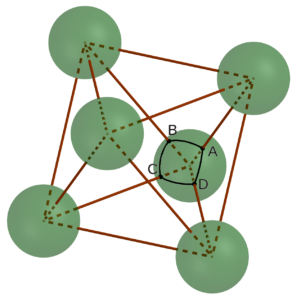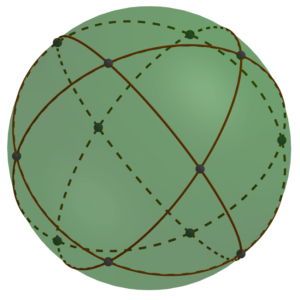04 Apr

The ease with which we could draw an equilateral triangle on the sphere naturally leads to wondering whether other constructions work out so nicely. For example, can we construct the square of points that would be needed to locate the holes for building a regular octahedron from styrofoam balls and sticks?Since the central angle between any two adjacent vertices of the square is again τ/6 = 60°, the sides of the square are the same length as the triangle’s sides in the previous construction. So let’s just look up the geometric construction of a square and use that on the sphere. Unfortunately, you immediately hit a snag: the first step is to “extend the line segment PQ.” And I don’t know of a really practical way to extend line segments on a sphere. You could try a flexible ruler, but it’s hard to get it lined up and to draw with it in place. Or you can make three equilateral triangles that all share the same vertex; then the side of the third one extends the original one. That’s fine in theory, but in practice it’s a lot of work and it’s easy for small errors to accumulate, leaving the sum of the three angles meeting at the vertex a bit different from 180° = τ/2.

So to make things much easier, we will use a special property of this particular square. As you can see in the picture, the regular octahedron can also be viewed as a square bipyramid. That tells us that the central angle between points A and C diagonally opposite on the square we want is τ/4 = 90°. And that in turn means that the diagonal of the square on the surface of the sphere is exactly 1.5 times as long as the side of the square. Contrast this to the situation of a square in the plane, where the diagonal is the much more computationally difficult √2 times the side of the square, but beware! This relationship does not hold for all squares on the surface of a sphere. Indeed, the ratio of diagonal to side length of spherical squares ranges from √2 to 2, and in fact, you can determine the side length of a spherical square from just that ratio (and the radius of the sphere).

But for the particular square we want, we do have this lovely relationship that the diagonal is 1.5 times the length of the side, and so we can find the other two corners just as we did for the equilateral triangle, simply using a thread of length d for one arc and 1.5d for the other arc. So building a regular octahedron should also be quite feasible.

We can go a bit further than this. If you play around with constructing these squares and triangles, you will find that alternating them completely covers the sphere.In other words, there are just twelve points on the surface of the sphere so that each one is a vertex of two equilateral triangles and two squares, alternating. That’s a pretty special structure, and it allows the existence of a very strong framework filling space called the oct-tet lattice consisting of alternating octahedra and tetrahedra.